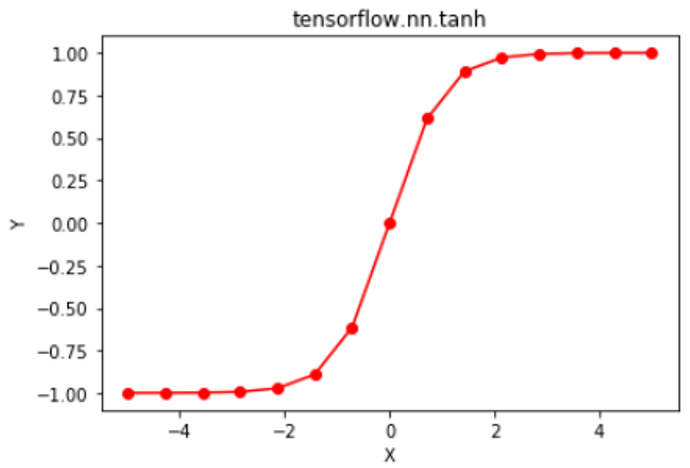Related Articles
Python | Tensorflow nn.tanh()
• Last Updated : 10 Dec, 2018

Tensorflow is an open-source machine learning library developed by Google. One of its applications is to develop deep neural networks.

The module `tensorflow.nn` provides support for many basic neural network operations.

One of the many activation functions is the hyperbolic tangent function (also known as tanh) which is defined as.

The hyperbolic tangent function outputs in the range (-1, 1), thus mapping strongly negative inputs to negative values. Unlike the sigmoid function, only near-zero values are mapped to near-zero outputs, and this solves the “vanishing gradients” problem to some extent. The hyperbolic tangent function is differentiable at every point and its derivative comes out to be. Since the expression involves the tanh function, its value can be reused to make the backward propagation faster.

Despite the lower chances of the network getting “stuck” when compared with the sigmoid function, the hyperbolic tangent function still suffers from “vanishing gradients”. Rectified Linear Unit (ReLU) can be used to overcome this problem.

The function `tf.nn.tanh()` [alias `tf.tanh`] provides support for the hyperbolic tangent function in Tensorflow.

Syntax: tf.nn.tanh(x, name=None) or tf.tanh(x, name=None)

Parameters:
x: A tensor of any of the following types: float16, float32, double, complex64, or complex128.
name (optional): The name for the operation.

Return : A tensor with the same type as that of x.

Code #1:

 `# Importing the Tensorflow library``import` `tensorflow as tf`` ` `# A constant vector of size 6``a ``=` `tf.constant([``1.0``, ``-``0.5``, ``3.4``, ``-``2.1``, ``0.0``, ``-``6.5``], dtype ``=` `tf.float32)`` ` `# Applying the tanh function and``# storing the result in 'b'``b ``=` `tf.nn.tanh(a, name ``=``'tanh'``)`` ` `# Initiating a Tensorflow session``with tf.Session() as sess:``    ``print``(``'Input type:'``, a)``    ``print``(``'Input:'``, sess.run(a))``    ``print``(``'Return type:'``, b)``    ``print``(``'Output:'``, sess.run(b))`

Output:

```Input type: Tensor("Const_2:0", shape=(6, ), dtype=float32)
Input: [ 1.        -0.5        3.4000001 -2.0999999  0.        -6.5      ]
Return type: Tensor("tanh_2:0", shape=(6, ), dtype=float32)
Output: [ 0.76159418 -0.46211717  0.9977749  -0.97045201  0.         -0.99999547]
```

Code #2: Visualization

 `# Importing the Tensorflow library``import` `tensorflow as tf`` ` `# Importing the NumPy library``import` `numpy as np`` ` `# Importing the matplotlib.pylot function``import` `matplotlib.pyplot as plt`` ` `# A vector of size 15 with values from -5 to 5``a ``=` `np.linspace(``-``5``, ``5``, ``15``)`` ` `# Applying the tanh function and``# storing the result in 'b'``b ``=` `tf.nn.tanh(a, name ``=``'tanh'``)`` ` `# Initiating a Tensorflow session``with tf.Session() as sess:``    ``print``(``'Input:'``, a)``    ``print``(``'Output:'``, sess.run(b))``    ``plt.plot(a, sess.run(b), color ``=` `'red'``, marker ``=` `"o"``) ``    ``plt.title(``"tensorflow.nn.tanh"``) ``    ``plt.xlabel(``"X"``) ``    ``plt.ylabel(``"Y"``) `` ` `    ``plt.show()`

Output:

```Input: [-5.         -4.28571429 -3.57142857 -2.85714286 -2.14285714 -1.42857143
-0.71428571  0.          0.71428571  1.42857143  2.14285714  2.85714286
3.57142857  4.28571429  5.        ]
Output: [-0.9999092  -0.99962119 -0.99842027 -0.99342468 -0.97284617 -0.89137347
-0.61335726  0.          0.61335726  0.89137347  0.97284617  0.99342468
0.99842027  0.99962119  0.9999092 ]
```Attention geek! Strengthen your foundations with the Python Programming Foundation Course and learn the basics.

To begin with, your interview preparations Enhance your Data Structures concepts with the Python DS Course.

My Personal Notes arrow_drop_up